Project tutorial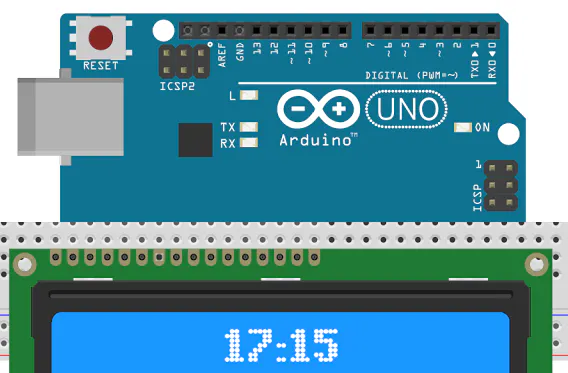Talking Clock

Using SD Card and 16x2 LCD module.

• 4,373 views
• 1 comment
• 10 respects

Components and supplies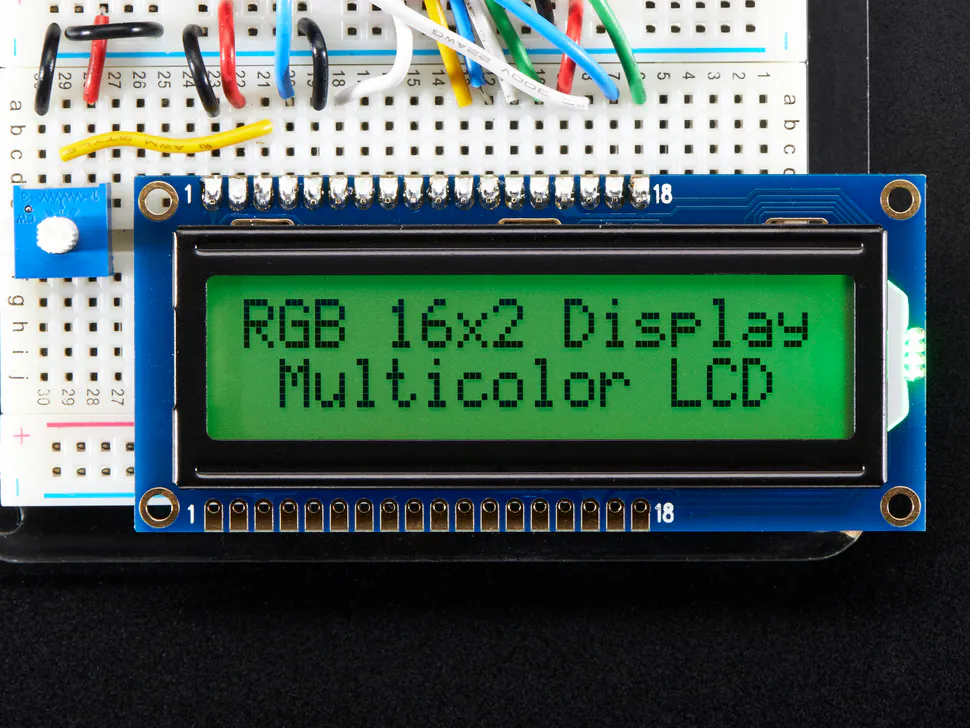Adafruit RGB Backlight LCD - 16x2
×1Arduino UNO & Genuino UNO
×1Resistor 1k ohm
×3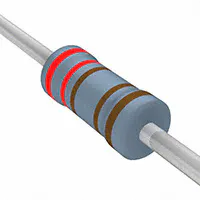Resistor 2.21k ohm
×3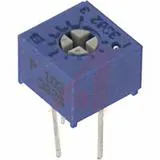Single Turn Potentiometer- 10k ohms
×1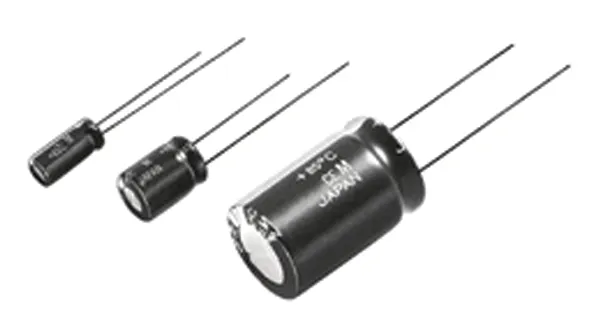Capacitor 22 µF
×2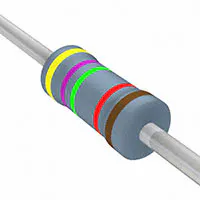Resistor 47.5k ohm
×1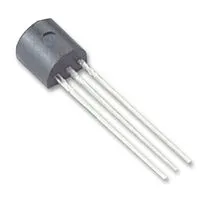General Purpose Transistor NPN
×1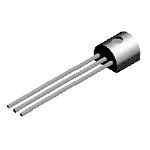General Purpose Transistor PNP
×1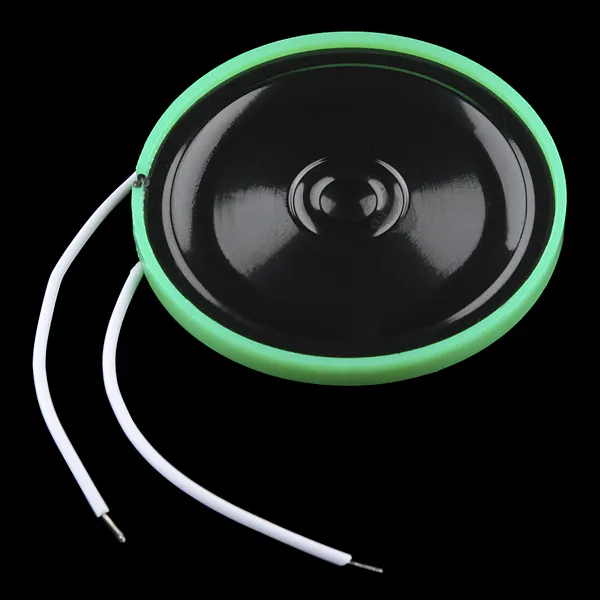Speaker: 0.25W, 8 ohms
×1SparkFun Pushbutton switch 12mm
×3
 SD Socket
×1

This Arduino has the basic knowledge to manage SD card and FAT32, it also runs 24 hours clock and generates audio signals.

The clock tells the time by playing back tracks from the SD. The SD is FAT32 formatted. The tracks are wave (.wav) files of 22.050 KHz, 8 bits, mono. Totally 27 files are recordings in language of your choice; 0,1,2...19,20,30,40,50,"hours","minutes",message

The SD card interface is in SPI mode. The ATMEGA328P generates audio using PWM.

The SD card has to be prepared this way:

• Files have to be 22.050 KHz, 8 bits, mono. With short names (max 8 characters).
• Format the card with FAT32. No folders or sub-folders.

Copy to the root folder the files one at a time and in this order; 0 to 19 (20 files),20,30,40,50,"hours","minutes","good day" (or any other message). Totally 27 files. If you make a mistake while copying the files to the SD it isn't enough to delete a file, you must start again with formatting the SD. The reason for loading the files one at a time is that if you load them together the PC sends them to the card in the order it finds fit, and when the code plays them it picks up the files without searching for their name.

Circuit Description

The SD Card supply is 3.3V (3-3.6V). The 3.3V and 5V are generated on the Arduino board. The PWM output is boosted by 2 transistors push-pull. The signal to the speaker is PWM of 62 KHz and the speaker outputs the modulated audio only. The speaker should be 8 ohms or higher. Digital outputs from the Arduino to the SD card use 1K and 2K resistors to reduce the 5V signals to 3.3V. The pins number are for ordinary SD card, for MicroSD the pins are different. Link between Arduino pins 3 and 5 connect 1000 Hz clock from timer 2 to timer 1, these timers generate 1 minute time base. LCD display and driver has 14 way connector, 10 connections are used, 4 bits data bus is selected. 10K pot adjusts the contrast.

To download wave files in English or more details see http://www.moty22.co.uk/talking_clock

Code

Talking ClockC/C++
/*
* sd_bell.ino
*
* Created: 5/06/2017 23:34:47
*  Author: moty22.co.uk
*/

#include <LiquidCrystal.h>

// initialize the library with the numbers of the interface pins
LiquidCrystal lcd(7, 8, A0, A1, A2, A3);

const int CS = 10;          // SD CS connected to digital pin PD4
const int mosi = 11;
const int clk = 13;
const int ampON = 9;          // led connected to digital pin PB0
const int audio = 6;     //output D6
const int oc2b = 3;     //1000Hz
const int t1 = 5;     //1000Hz
const int talk = 2;     //talk pushbutton
const int minutes = A4;     //minutes advance pushbutton
const int hours = A5;     //hours pushbutton
int talkPB=1;         // talk pushbutton status
int minutesPB=1;         // minutes pushbutton status
int hoursPB=1;         // hours pushbutton status

unsigned long loc,BootSector, RootDir, SectorsPerFat, RootDirCluster, DataSector, FileCluster, FileSize;  //
unsigned int BytesPerSector, ReservedSectors, card;
unsigned char fn=1, sdhc=0, SectorsPerCluster, Fats;
unsigned char minute=0, hour=0;

void setup() {
// put your setup code here, to run once:
pinMode(CS, OUTPUT);
pinMode(mosi, OUTPUT);
pinMode(clk, OUTPUT);
pinMode(ampON, OUTPUT);
pinMode(talk, INPUT_PULLUP);
pinMode(minutes, INPUT_PULLUP);
pinMode(hours, INPUT_PULLUP);

lcd.begin(16, 2);   // set up the LCD's number of columns and rows:

//spi init
SPCR = _BV(SPE) | _BV(MSTR) | _BV(SPR0) | _BV(SPR1);  // Enable spi, Master, set clock rate fck/64
SPSR = _BV(SPI2X);    //set clock rate fck/64 = 250KHz

//PWM Timer0
OCR0A = 0;
TCCR0A = _BV(COM0A1) | _BV(WGM01) | _BV(WGM00);  //output in phase, fast PWM mode
TCCR0B = _BV(CS00); // 16MHz/256 = 62.5KHz
pinMode(audio, OUTPUT);

//1000 Hz - timer2
OCR2A =249;
OCR2B = 125;
TCCR2A = _BV(COM2B1) | _BV(COM2B0) | _BV(WGM21) | _BV(WGM20);  //output B in phase, fast PWM mode
TCCR2B = _BV(WGM22) | _BV(CS22); // set prescaler to 64 and start the timer
pinMode(oc2b, OUTPUT);

//60 sec - timer1
// OCR1A = 0xEA5F;
TCCR1A = _BV(WGM10) | _BV(WGM11) | _BV(COM1A0); //
TCCR1B = _BV(WGM12) | _BV(WGM13) | _BV(CS11) | _BV(CS12);  // input T1, PWM mode

digitalWrite(CS, HIGH);
//digitalWrite(ampON, LOW);

InitSD();
if(sdhc){card = 1;}else{card=512;}  //SD or SDHC
fat();
if(BytesPerSector!=512){error();}

}

void loop()
{

if(talkPB==LOW)
{
if(hour < 21) {fn=hour; play();}
else if(hour == 20) {fn=20; play();}
else if(hour > 20) {fn=20; play(); fn=hour-20; play();}
fn=24; play();     //read "hours"

if(minute < 21) {fn=minute; play();}
else if(minute == 20) {fn=20; play();}
else if(minute > 20 && minute < 30) {fn=20; play(); fn=minute-20; play();}
else if(minute == 30) {fn=21; play();}
else if(minute > 30 && minute < 40) {fn=21; play(); fn=minute-30; play();}
else if(minute == 40) {fn=22; play();}
else if(minute > 40 && minute < 50) {fn=22; play(); fn=minute-40; play();}
else if(minute == 50) {fn=23; play();}
else if(minute > 50 && minute < 60) {fn=23; play(); fn=minute-50; play();}
fn=25; play();    //read "minutes"
fn=26; play();    //read message
OCR0A=0;    //audio off

}

if(minutesPB==LOW)
{
minute++;
if(minute>59) {minute=0;}
display();
wait();
}

if(hoursPB==LOW)
{
hour++;
if(hour>23) {hour=0;}
display();
wait();
}

if(TIFR1 & _BV(OCF1A))   //1 minute elapsed
{
OCR1A = 0xEA5F;  //59999 counts = 1 minute
TIFR1 = _BV(OCF1A);  //TMR1 interrupt reset

minute++;
if(minute>59) {minute=0; hour++;}
if(hour>23) hour=0;
display();

}
}

//find the file and play it
void play(void)
{
if(!sdhc){fn += 1;}  //if not SDHC first file = 1
if(fn > 15)
{
fn -=16; file(fn*32+20,1);    //32 bytes per file descriptor at offset of 20
}
else
{
file(fn*32+20,0);    //32 bytes per file descriptor at offset of 20
}
loc=(1 + (DataSector) + (unsigned long)(FileCluster-2) * SectorsPerCluster) * card ;
}

//LCD update
void display(void)
{
lcd.setCursor(5, 0); // top left
if(hour<10)lcd.print(" ");
lcd.print(hour);
lcd.print(":");
if(minute<10)lcd.print("0");
lcd.print(minute);
}

//SD error display
void error(void)
{
lcd.setCursor(0, 1); // bottom left
lcd.print("SD ERROR");
lcd.setCursor(0, 0); // top left
}

//1 sec delay
void wait(void)
{
unsigned long i; //,j
for(i=0;i<100000;i++)
{
digitalWrite(ampON, LOW);
}
}

{
unsigned int i,r;
digitalWrite(CS, LOW);
r = Command(18,loc,0xFF); //read multi-sector
if(r != 0) error();     //if command failed error();

while(FileSize--)
{
while(spi(0xFF) != 0xFE); // wait for first byte
for(i=0;i<512;i++){
// 3 rounds of timer 0 = total of time to get 22 khz
while (!(TIFR0 & _BV(OCF0A))){}
TIFR0 = _BV(OCF0A);  //TMR0 interrupt reset
while (!(TIFR0 & _BV(OCF0A))){}
TIFR0 = _BV(OCF0A);  //TMR0 interrupt reset
while (!(TIFR0 & _BV(OCF0A))){}

OCR0A=spi(0xFF);    //write byte to PWM
TIFR0 = _BV(OCF0A);  //TMR0 interrupt reset
}
spi(0xFF);  //discard of CRC
spi(0xFF);
}

Command(12,0x00,0xFF);  //stop transmit
spi(0xFF);
spi(0xFF);
digitalWrite(CS, HIGH);
spi(0xFF);
}

void file(unsigned int offset, unsigned char sect)  //find files
{
unsigned int r,i;
unsigned char fc, fs; //

digitalWrite(CS, LOW);
r = Command(17,(RootDir+sect)*card,0xFF);   //read boot-sector for info from file entry
if(r != 0) error();     //if command failed

while(spi(0xFF) != 0xFe); // wait for first byte
for(i=0;i<512;i++){
if(i==offset){fc=spi(0xFF);}
else if(i==offset+1){fc=spi(0xFF);}
else if(i==offset+6){fc=spi(0xFF);}
else if(i==offset+7){fc=spi(0xFF);}

else if(i==offset+8){fs=spi(0xFF);}
else if(i==offset+9){fs=spi(0xFF);}
else if(i==offset+10){fs=spi(0xFF);}
else if(i==offset+11){fs=spi(0xFF);}
else{spi(0xFF);}

}
spi(0xFF);  //discard of CRC
spi(0xFF);
digitalWrite(CS, HIGH);
spi(0xFF);
FileCluster = fc | ( (unsigned long)fc << 8 ) | ( (unsigned long)fc << 16 ) | ( (unsigned long)fc << 24 );
FileSize = fs | ( (unsigned long)fs << 8 ) | ( (unsigned long)fs << 16 ) | ( (unsigned long)fs << 24 );
FileSize = FileSize/512-1;    //file size in sectors
}

void fat (void)
{
unsigned int r,i;
unsigned char pfs,bps1,bps2,rs1,rs2,spf,rdc; //pfs=partition first sector ,de1,de2,spf1,d

digitalWrite(CS, LOW);
r = Command(17,0,0xFF);   //read MBR-sector
if(r != 0) error();     //if command failed

while(spi(0xFF) != 0xFe); // wait for first byte
for(i=0;i<512;i++){
if(i==454){pfs=spi(0xFF);} //pfs=partition first sector
else if(i==455){pfs=spi(0xFF);}
else if(i==456){pfs=spi(0xFF);}
else if(i==457){pfs=spi(0xFF);}
else{spi(0xFF);}

}
spi(0xFF);  //discard of CRC
spi(0xFF);
digitalWrite(CS, HIGH);
spi(0xFF);
//convert 4 bytes to long int
BootSector = pfs | ( (unsigned long)pfs << 8 ) | ( (unsigned long)pfs << 16 ) | ( (unsigned long)pfs << 24 );

digitalWrite(CS, LOW);
r = Command(17,BootSector*card,0xFF);   //read boot-sector
if(r != 0) error();     //if command failed

while(spi(0xFF) != 0xFe); // wait for first byte
for(i=0;i<512;i++){

if(i==11){bps1=spi(0xFF);} //bytes per sector
else if(i==12){bps2=spi(0xFF);}
else if(i==13){SectorsPerCluster=spi(0xFF);}
else if(i==14){rs1=spi(0xFF);}
else if(i==15){rs2=spi(0xFF);}
else if(i==16){Fats=spi(0xFF);} //number of FATs
else if(i==36){spf=spi(0xFF);}
else if(i==37){spf=spi(0xFF);}
else if(i==38){spf=spi(0xFF);}
else if(i==39){spf=spi(0xFF);}
else if(i==44){rdc=spi(0xFF);}
else if(i==45){rdc=spi(0xFF);}
else if(i==46){rdc=spi(0xFF);}
else if(i==47){rdc=spi(0xFF);}
else{spi(0xFF);}

}
spi(0xFF);  //discard of CRC
spi(0xFF);
digitalWrite(CS, HIGH);
spi(0xFF);

BytesPerSector = bps1 | ( (unsigned int)bps2 << 8 );
ReservedSectors = rs1 | ( (unsigned int)rs2 << 8 ); //from partition start to first FAT
RootDirCluster = rdc | ( (unsigned long)rdc << 8 ) | ( (unsigned long)rdc << 16 ) | ( (unsigned long)rdc << 24 );
SectorsPerFat = spf | ( (unsigned long)spf << 8 ) | ( (unsigned long)spf << 16 ) | ( (unsigned long)spf << 24 );
DataSector = BootSector + (unsigned long)Fats * (unsigned long)SectorsPerFat + (unsigned long)ReservedSectors;  // + 1
RootDir = (RootDirCluster -2) * (unsigned long)SectorsPerCluster + DataSector;
}

unsigned char spi(unsigned char data)   // send character over spi
{
SPDR = data;  // Start transmission
while(!(SPSR & _BV(SPIF)));   // Wait for transmission to complete
return SPDR;    // received byte

}
//assemble a 32 bits command
char Command(unsigned char frame1, unsigned long adrs, unsigned char frame2 )
{
unsigned char i, res;
spi(0xFF);
spi((frame1 | 0x40) & 0x7F);  //first 2 bits are 01
spi((adrs & 0xFF000000) >> 24);   //first of the 4 bytes address
spi((adrs & 0x00FF0000) >> 16);
spi((adrs & 0x0000FF00) >> 8);
spi(frame2 | 1);        //CRC and last bit 1

for(i=0;i<10;i++) // wait for received character
{
res = spi(0xFF);
if(res != 0xFF)break;
}
return res;
}

void InitSD(void)
{
unsigned char i,r;

digitalWrite(CS, HIGH);
for(i=0; i < 10; i++)spi(0xFF);   // min 74 clocks
digitalWrite(CS, LOW);      // Enabled for spi mode

i=100;  //try idle state for up to 100 times
while(Command(0x00,0,0x95) !=1 && i!=0)
{
digitalWrite(CS, HIGH);
spi(0xFF);
digitalWrite(CS, LOW);
i--;
}
if(i==0)  error(); //idle failed

if (Command(8,0x01AA,0x87)==1){         //check card is 3.3V
r=spi(0xFF); r=spi(0xFF); r=spi(0xFF); r=spi(0xFF);   //rest of R7
if ( r == 0x01 && r == 0xAA ){    //Vdd OK (3.3V)

//Command(59,0,0xFF);   //CRC off
Command(55,0,0xFF);
while(Command(41,0x40000000,0xFF)){Command(55,0,0xFF);}   //ACMD41 with HCS bit
}
}else{error();}

if (Command(58,0,0xFF)==0){   //read CCS in the OCR - SD or SDHC
r=spi(0xFF); r=spi(0xFF); r=spi(0xFF); r=spi(0xFF);   //rest of R3
sdhc=r & 0x40;
//if(r & 0x40)sdLED=1;
}
SPCR &= ~(_BV(SPR1));  // set clock rate fck/8 = 2MHz
digitalWrite(CS, HIGH);
}

SchematicsTalking Clock 2 - New Version (Bilingual: EN-PT)

Project tutorial by LAGSILVA

• 11,060 views
• 41 respects

Adjusting Dual Clock using DS3231 on 1.8" ST7735 Display

Project showcase by FLORICA Tudor-Nicusor

• 6,209 views
• 15 respects

Digital Clock

Project in progress by Team SharKode

• 7,887 views
• 30 respects

The Talking Alarm Clock

Project tutorial by Abe-Z

• 2,507 views
• 9 respects

Alarm Clock and Timer Working Standalone

Project showcase by ozyRonald

• 11,906 views